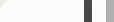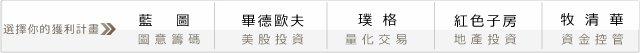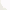## 凱利賭徒的另一波高潮：Optimal f 資金控管!

1. 凱利討論的是傳統賭局的最佳化賭法，傳統賭局有"固定的"機率跟賠率，只要期望值是正的，就可套用凱利公式下注，而實際交易卻不是這樣。(沒有固定的機率跟賠率)

2. 賭場裡的賭局是用籌碼下注，籌碼有最小單位，可能是\$SGD5元、\$USD5元、\$MOP10元、\$KRW1000元....等(交易也有口數、股價等基本單位)。而凱利卻是比例下注，當凱利下注比例小於籌碼的最小單位，或是下注金額無法用最小單位組成，將發生無法下注的窘境!

1. 在這10筆交易裡，有5次獲利，5次虧損。勝率50%。

2. 平均賺 (19+8+10+20+12)/5 = 13.8；平均賠 (-7-11-5-3-15)/5 = -8.2，

[p(1+b)-1]/b = [50%(1+1.68)-1]/1.68 =  20.24%

#### 初窺Vince's 最佳化f (Optimal f)

Vince很巧妙的採用Kelly的手法，但避開固定機率與賠率的限制。

HPR1 = 1+f*(-損益/最大虧損) = 1+f*(-19/-15)

HPR2 = 1+f*(-損益/最大虧損) = 1+f*(-8/-15)

HPR3 = 1+f*(-損益/最大虧損) = 1+f*(-(-7)/-15)
...
...

HPR9 = 1+f*(-損益/最大虧損) = 1+f*(-12/-15)
...

HPR10 = 1+f*(-損益/最大虧損) = 1+f*(-(-15)/-15) = 1 - f

Geo. Mean = (HPR1*HPR2*...*HPR10)^(1/10)

HPR連乘積與幾何平均數的圖形如下：

#### 然道凱利錯了嗎?

Vince用曾經發生過的最大虧損當作每次輸贏可能的最大損失(當作分母)，每次的損益當作分子，取代傳統賭局裡的賠率b，並計算其幾何平均數。如此避開凱利固定機率與陪率的限制。

PS: 截圖模特兒也叫凱利(Kelly Monaco)，牧清華真的很愛Kelly~

#### 0 意見: